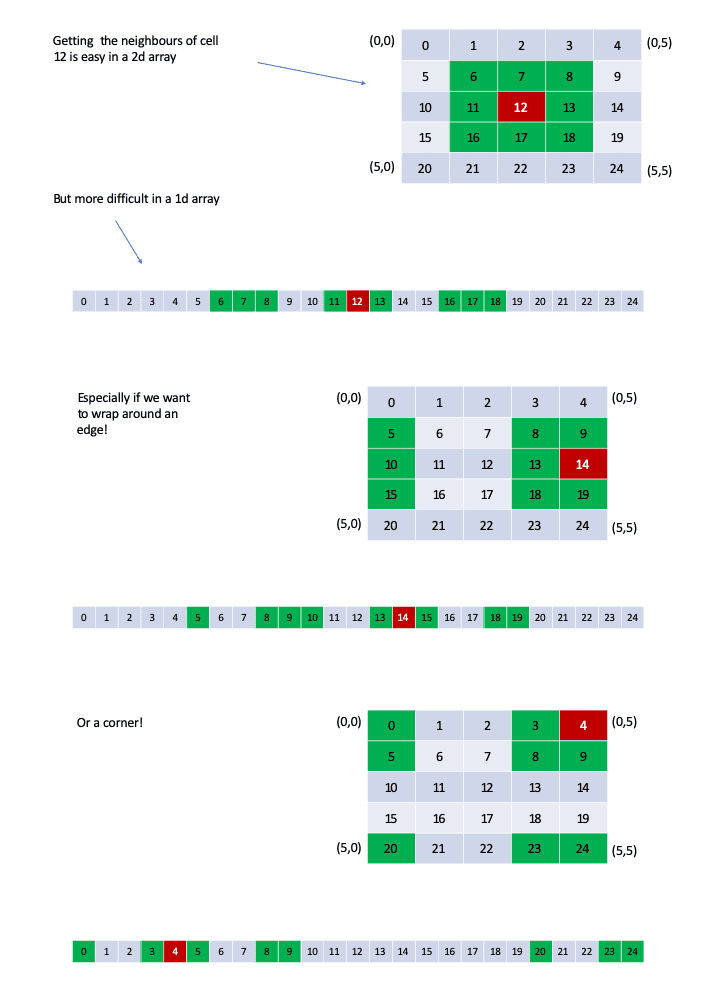# grid-neighbors-1d

Get the 8 closest neighbors of a cell in a 2d grid when flattened to a 1d array

## Usage no npm install needed!

``````<script type="module">
import gridNeighbors1d from 'https://cdn.skypack.dev/grid-neighbors-1d';
</script>``````

# grid-neighbors-1d

Get the 8 closest neighbors of a grid with edge wrapping from a 1d array

## Why?## Usage

Get the neighbors of cell 12 in a 5x6 grid:

``````const gn = require('grid-neighbors-1d');
const neighbors = gn(12, 5, 6);
console.log(neighbors); // [7, 8, 13, 18, 17, 16, 11, 6] - clockwise from north
``````

grid-neighbors returns an 0-based array of indexes of the cell immediately to the north of the chosen cell, then clockwise around the remaining cells:

• neighbors = north neighbor
• neighbors = north east neighbor
• neighbors = east neighbor
• neighbors = south east neighbor
• neighbors = south neighbor
• neighbors = south west neighbor
• neighbors = west neighbor
• neighbors = north west neighbor